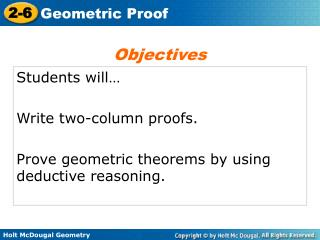DownloadDownload PresentationStudents will… Write two-column proofs. Prove geometric theorems by using deductive reasoning.

Students will… Write two-column proofs. Prove geometric theorems by using deductive reasoning.

Télécharger la présentationStudents will… Write two-column proofs. Prove geometric theorems by using deductive reasoning.

- - - - - - - - - - - - - - - - - - - - - - - - - - - E N D - - - - - - - - - - - - - - - - - - - - - - - - - - -
Presentation Transcript

1. Objectives Students will… Write two-column proofs. Prove geometric theorems by using deductive reasoning.

2. Warm Up Determine whether each statement is true or false. If false, give a counterexample. 1.It two angles are complementary, then they are not congruent. 2. If two angles are congruent to the same angle, then they are congruent to each other. 3. Supplementary angles are congruent. false; 45° and 45° true false; 60° and 120°

3. Vocabulary theorem two-column proof

4. Definitions • Postulates • Properties • Theorems Conclusion Hypothesis When writing a proof, justify each logical step with a reason. Symbols and abbreviations can be used, but must be clear so the viewer understands.

5. Example 1: Writing Justifications Write a justification for each step, given that A and Bare supplementary and mA = 45°. 1. Aand Bare supplementary. mA = 45° Given information 2. mA+ mB= 180° Def. of supp s 3. 45°+ mB= 180° Subst. Prop of = Steps 1, 2 4. 45°+ mB - 45°= 180° - 45° Subtr. Prop of = 4. mB= 135° Subst. Prop of =

6. Helpful Hint When a justification (reason) is based on more than the previous step, note this after the reason, as in Example 1 Step 3. theorem - any statement that you can prove. - -Once proven, you can use it as a reason in later proofs. Postulates are accepted as true but can't be proven!

7. A geometric proof can begin with Given(Hypothesis) and Prove(Conclusion) statements. In a two-column proof, list the steps of the proof in the left column and justifications(reasons) in the right column.

8. Example 2: Completing a Two-Column Proof Fill in the blanks to complete the two-column proof. Given: XY Prove: XY  XY Reflex. Prop. of =

9. Helpful Hint If a diagram for a proof is not provided, draw your own and mark the given information on it. But do not mark the information in the Prove statement on it.

10. Example 3: Writing a Two-Column Proof from a Plan Use the given plan to write a two-column proof. Given: 1 and 2 are supplementary, and 1  3 Prove: 3 and 2 are supplementary. Plan: Use the definitions of supplementary and congruent angles and substitution to show that m3 + m2 = 180°.By the definition of supplementary angles, 3 and 2are supplementary.

11. Example 3 Continued Given 1 and 2 are supplementary. 1  3 m1+ m2 = 180° Def. of supp. s m1= m3 Def. of s Subst. m3+ m2 = 180° Def. of supp. s 3 and 2 are supplementary

12. Lesson Quiz: Part I Write a justification for each step, given that mABC= 90° and m1= 4m2. 1. mABC= 90° and m1= 4m2 2. m1+ m2 = mABC 3. 4m2 + m2 = 90° 4. 5m2= 90° 5. m2= 18° Given  Add. Post. Subst. Simplify Div. Prop. of =.

13. Write a justification for each step, given that B is the midpoint of AC and ABEF. 1. Bis the midpoint of AC. 2. AB BC 3. AB EF 4. BC EF Check It Out! Example 1 Given information Def. of mdpt. Given information Trans. Prop. of 

14. Lesson Quiz: Part II 2. Use the given plan to write a two-column proof. Given: 1, 2 , 3, 4 Prove: m1 + m2 = m1 + m4 Plan: Use the linear Pair Theorem to show that the angle pairs are supplementary. Then use the definition of supplementary and substitution. 1. 1 and 2 are supp. 1 and 4 are supp. 1. Linear Pair Thm. 2. Def. of supp. s 2. m1+ m2 = 180°, m1+ m4 = 180° 3. Subst. 3. m1+ m2 = m1+ m4

15. Check It Out! Example 2 Fill in the blanks to complete a two-column proof of one case of the Congruent Supplements Theorem. Given: 1 and 2 are supplementary, and 2 and 3 are supplementary. Prove: 1  3 Proof: • 1 and 2 are supp., and 2 and 3 are supp. b. m1 + m2 = m2 + m3 c. Subtr. Prop. of = d. 1  3

16. Check It Out! Example 3 Use the given plan to write a two-column proof if one case of Congruent Complements Theorem. Given: 1 and 2 are complementary, and 2 and 3 are complementary. Prove: 1  3 Plan: The measures of complementary angles add to 90° by definition. Use substitution to show that the sums of both pairs are equal. Use the Subtraction Property and the definition of congruent angles to conclude that 1 3.

17. Check It Out! Example 3 Continued Given 1 and 2 are complementary. 2 and 3 are complementary. m1+ m2 = 90° m2+ m3 = 90° Def. of comp. s m1+ m2 = m2+ m3 Subst. Reflex. Prop. of = m2= m2 m1 = m3 Subtr. Prop. of = 1  3 Def. of  s